• 线性变换是灰度变换的一种，图像的灰度变换通过建立灰度映射来调整源图像的灰度从而达到图像增强的目的。  其公式可以表示为y(x, y) = k * x(x, y) + b;其中y(x, y)表示目标像素值，x(x, y)表示源像素值，k表示...
线性变换是灰度变换的一种，图像的灰度变换通过建立灰度映射来调整源图像的灰度从而达到图像增强的目的。
其公式可以表示为y(x, y) = k * x(x, y) + b;其中y(x, y)表示目标像素值，x(x, y)表示源像素值，k表示斜率，b表示截距。

1）当k>1时，可用于增加图像的对比度。图像的像素值在变换后全部增大，整体显示效果被增强。
2）当k=1时，常用于调节图像亮度。
3）当0<k<1时，效果与k>1时刚刚相反，图像的对比度和整体效果都被削弱。
4）当k<0时，源图像较亮的区域变暗，而较暗的区域会变亮。此时可以使函数中的k=-1，d=255让图像实现反色效果。
代码如下：

[cpp]
view plaincopyprint?

/******************************************************************************
*   作用:     线性变换函数 *   参数: pDst     输出图像的像素数组
*   参数: pSrc     原始图像的像素数组 *   参数: nWidth   原始图像宽度
*   参数: nHeight  原始图像高度 *   参数: slope      线性函数的斜率
*   参数: inter      线性函数的截距 *   备注: 此函数对于彩色图同样适用 ******************************************************************************/
int LineTrans(BYTE* pDst,
BYTE* pSrc, int nWidth,
int nHeight, double slope,
double inter)  {      if (!pSrc || !pDst)
{          return EXIT_FAILURE;
}        // 灰度映射表      BYTE map;
// 保存运算后的临时值
double dTemp;      int i, j;
for (i = 0; i < 256; i++)
{          // 计算当前像素变换后的值          dTemp = slope * i + inter;            // 如果超界则修改其值
if (dTemp < 0)              dTemp = 0.0;          else if (dTemp > 255)
dTemp = 255;            // 四舍五入
map[i] = int(dTemp + 0.5);
}        // 线性变换后的值直接在映射表中查找
for (i = 0; i < nWidth * nHeight; i++)
{             for (j = 0; j < 4; j++)
pDst[i*4 + j] = map[pSrc[i*4 + j]];      }      return EXIT_SUCCESS;
}


展开全文图像处理 算法 byte
• 线性变换和伽马变换C++实现
1.灰度线性变换：F(x)=a*f(x)+b
int IMGChange(IplImage *src,IplImage *dest, float slope,float intercept)
{
if(NULL == src)
return -1;
if(src->nChannels!=1 || dest->nChannels!=1)
{
cout<<"It's not gray image!"<<endl;
return -1;
}
float gray = 0;
for(int i = 0;i < src->height;i++){
for(int j = 0; j < src->width; j++){

gray = (float)src->imageData[src->widthStep * i + j ];
gray = slope*gray + intercept;
dest->imageData[dest->widthStep * i + j ]=gray;
}
}
return 0;
}
当slope=-1   intercept=255即为图像反转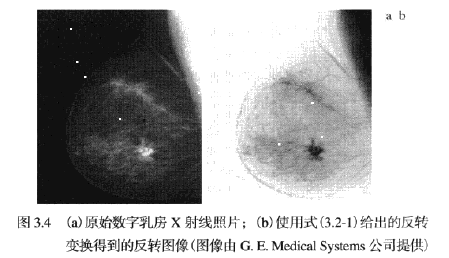2.伽马变换，s=c*（r）γ，可用于图像增强等预处理。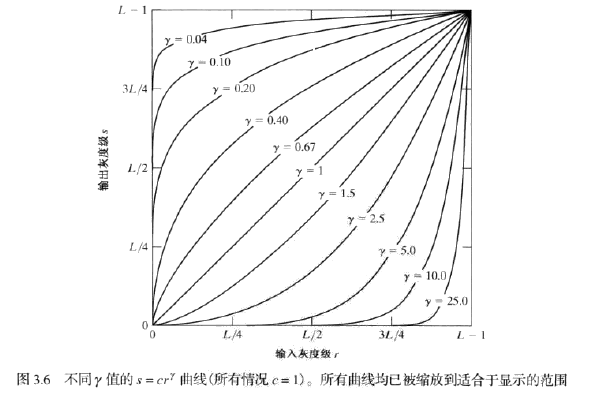int IMGgammaChange(IplImage *src,IplImage *dest, double gamma,double comp)
{
if(NULL == src)
return -1;
if(src->nChannels!=1 || dest->nChannels!=1)
{
cout<<"It's not gray image!"<<endl;
return -1;
}
double gray = 0;
for(int i = 0;i < src->height;i++){
for(int j = 0; j < src->width; j++){

gray = (double)src->imageData[src->widthStep * i + j ];
gray =pow((gray+comp)/255.0,gamma)*255;
dest->imageData[dest->widthStep * i + j ]=gray;
}
}
return 0;
}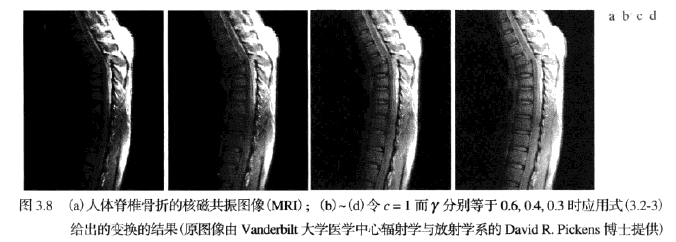﻿﻿
﻿﻿

展开全文• 图像灰度线性变换 文章目录1 概念2 原理3 作用4 Matlab实现5 OpenCV实现6 效果图6.1 效果图7 讨论 1 概念   灰度线性变换是一种灰度变换，通过建立灰度映射来调整源图像的灰度，达到图像增强的目的。灰度映射...
图像灰度线性变换
文章目录1 概念2 原理3 作用4 Matlab实现5 OpenCV实现6 效果图6.1 效果图7 讨论
1 概念
灰度线性变换是一种灰度变换，通过建立灰度映射来调整源图像的灰度，达到图像增强的目的。灰度映射通常使用灰度变换曲线来表示。
2 原理
灰度线性变换就是将图像的像素值通过指定的线性函数进行变换，以此增强或减弱图像的灰度。灰度线性变换的公式是常见的一维线性函数：
$g(x,y) = k \cdot f(x,y) + b$
设$x$为原始灰度值，则变换后的灰度值$y$为：
$y = k \cdot x + b \dots\dots(0 \leq y \leq 255)$
$k$表示直线的斜率，即倾斜程度，$b$表示线性函数在$y$轴的截距。
3 作用

$k$ $b$取值
意义

$k>1$
增大图像的对比度，图像的像素值在变换后全部增大，整体效果被增强

$k=1$
通过调整$b$，实现对图像亮度的调整

$0 < k < 1$
图像的对比度被削弱

$k<0$
原来图像亮的区域变暗，原来图像暗的区域变亮

4 Matlab实现
clc;
clear;
close all;

% 对灰度图进行灰度线性变换
ori_img = rgb2gray(ori_img);
[oriHist,oriX] = imhist(ori_img);

k = 1.25;
d = 0;
gray1 = ori_img * k + d;
[g1Hist,g1X] = imhist(gray1);

k = 1;
d = 50;
gray2 = ori_img * k + d;
[g2Hist,g2X] = imhist(gray2);

k = 0.5;
d = 0;
gray3 = ori_img * k + d;
[g3Hist,g3X] = imhist(gray3);

k = -1;
d = 255;
ori_ = im2double(ori_img);
gray4 = ori_ * k + 1.0;
[g4Hist,g4X] = imhist(gray4);

figure(1),subplot(1,2,1),imshow(ori_img),title('原图');subplot(1,2,2),imshow(gray1),title('k>0 d=0');
figure(2),subplot(1,2,1),stem(oriX,oriHist),title('原图直方图');subplot(1,2,2),stem(g1X,g1Hist),title('k>0 d=0直方图');
figure(3),subplot(1,2,1),imshow(ori_img),title('原图');subplot(1,2,2),imshow(gray2),title('k=1 d=50');
figure(4),subplot(1,2,1),stem(oriX,oriHist),title('原图直方图');subplot(1,2,2),stem(g2X,g2Hist),title('k=1 d=50直方图');
figure(5),subplot(1,2,1),imshow(ori_img),title('原图');subplot(1,2,2),imshow(gray3),title('k=0.5 d=0');
figure(6),subplot(1,2,1),stem(oriX,oriHist),title('原图直方图');subplot(1,2,2),stem(g3X,g3Hist),title('k=0.5 d=0直方图');
figure(7),subplot(1,2,1),imshow(ori_img),title('原图');subplot(1,2,2),imshow(gray4),title('k=-1 d=255');
figure(8),subplot(1,2,1),stem(oriX,oriHist),title('原图直方图');subplot(1,2,2),stem(g4X,g4Hist),title('k=-1 d=255直方图');

5 OpenCV实现
#include <iostream>
#include <string>

#include "../include/opencv400/opencv2/opencv.hpp"
#include "windows.h"

std::string g_CurrentDirectory;
void SetCurrentDirectoryToExePath()
{
HMODULE hExe = GetModuleHandleA(NULL);
char nameBuf[MAX_PATH] = { 0 };
GetModuleFileNameA(hExe, nameBuf, MAX_PATH);
std::string sName(nameBuf);
sName = sName.substr(0, sName.rfind('\\'));
SetCurrentDirectoryA(sName.c_str());
g_CurrentDirectory = sName;
}

void calcHist1D(cv::Mat& input, cv::Mat& output)
{
int channels[] = { 0 };
int histsize[] = { 256 };
float grayRnage[] = { 0,256 };
const float* ranges[] = { grayRnage };
cv::MatND hist;
cv::calcHist(&input, 1, channels, cv::Mat(), hist, 1, histsize, ranges);

double maxVal = 0;
cv::minMaxLoc(hist, 0, &maxVal, 0, 0);

int scale = 10;
output = cv::Mat::zeros(500, 257 * 5, CV_8UC3);

std::cout << "-----------------------------------" << std::endl;
for (int i = 0; i < histsize; i++)
{
float binVal = hist.at<float>(i, 0);
std::cout <<i <<" "<< binVal << std::endl;
int intensity = cvRound(binVal * 500 / maxVal);
rectangle(output, cv::Point(i * 5, 500 - intensity),
cv::Point((i + 1) * 5, 500),
cv::Scalar::all(255),
-1);
}

}

int main()
{
SetCurrentDirectoryToExePath();

cv::Mat gray_img;
cv::cvtColor(ori_img, gray_img, cv::COLOR_BGR2GRAY);
//gray_img.convertTo(gray_img, CV_32FC1, 1.0 / 255);
cv::namedWindow("灰度图");
cv::imshow("灰度图", gray_img);

cv::Mat grayHist;
calcHist1D(gray_img, grayHist);
cv::imshow("hist", grayHist);

float k = 1.25;
int d = 0;
cv::Mat g1 = gray_img * k + d;
cv::Mat g1Hist;
calcHist1D(g1, g1Hist);
cv::imshow("g1", g1);
cv::imshow("g1Hist", g1Hist);

k = 1;
d = 30;
cv::Mat g2 = gray_img * k + d;
cv::Mat g2Hist;
calcHist1D(g2, g2Hist);
cv::imshow("g2", g2);
cv::imshow("g2Hist", g2Hist);

k = 0.5;
d = 0;
cv::Mat g3 = gray_img * k + d;
cv::Mat g3Hist;
calcHist1D(g3, g3Hist);
cv::imshow("g3", g3);
cv::imshow("g3Hist", g3Hist);

k = -1;
d = 255;
cv::Mat g4 = gray_img * k + d;
cv::Mat g4Hist;
calcHist1D(g4, g4Hist);
cv::imshow("g4", g4);
cv::imshow("g4Hist", g4Hist);

cv::waitKey();
return 0;
}


6 效果图
原图6.1 效果图
k> 1 b=0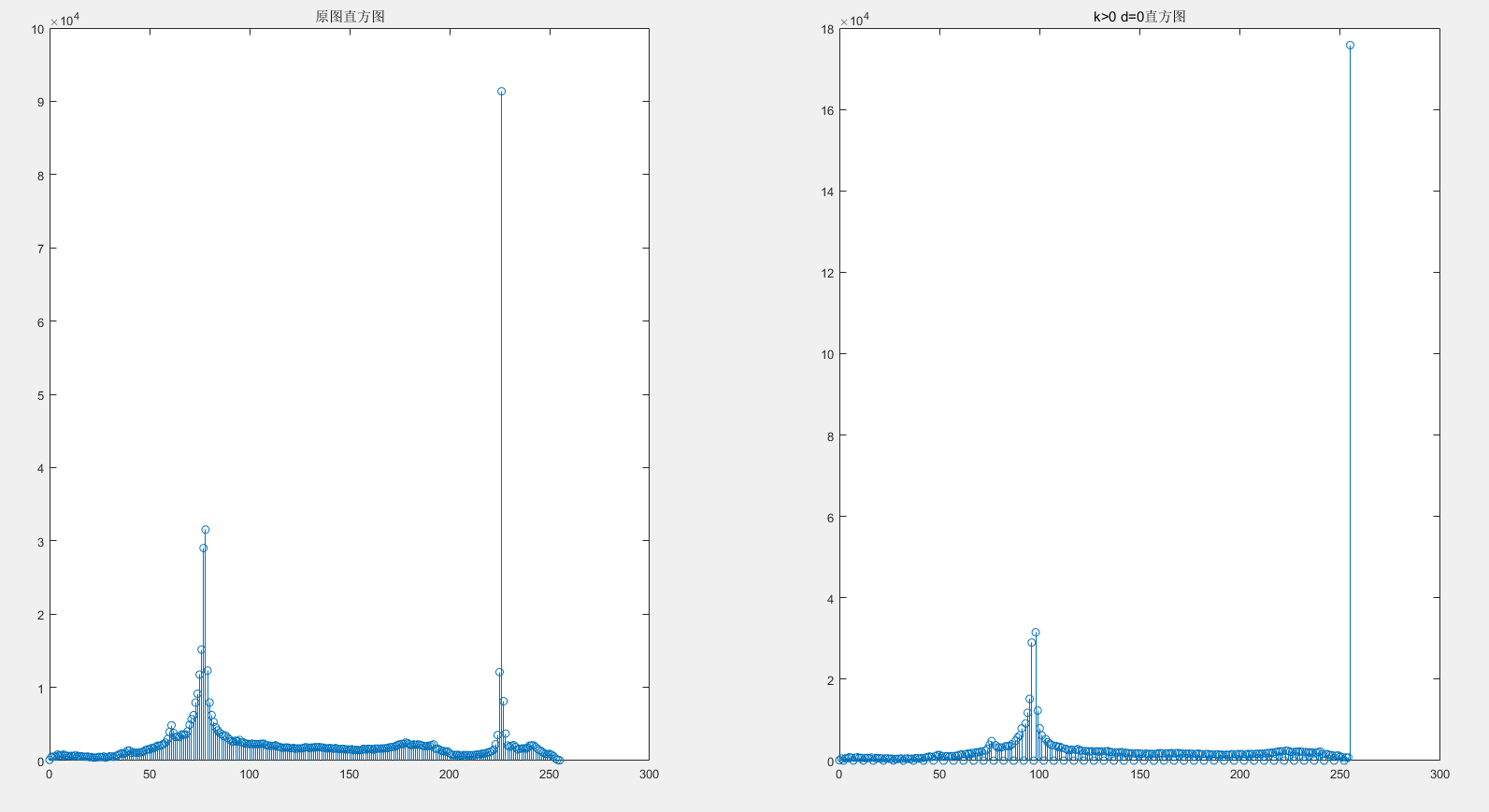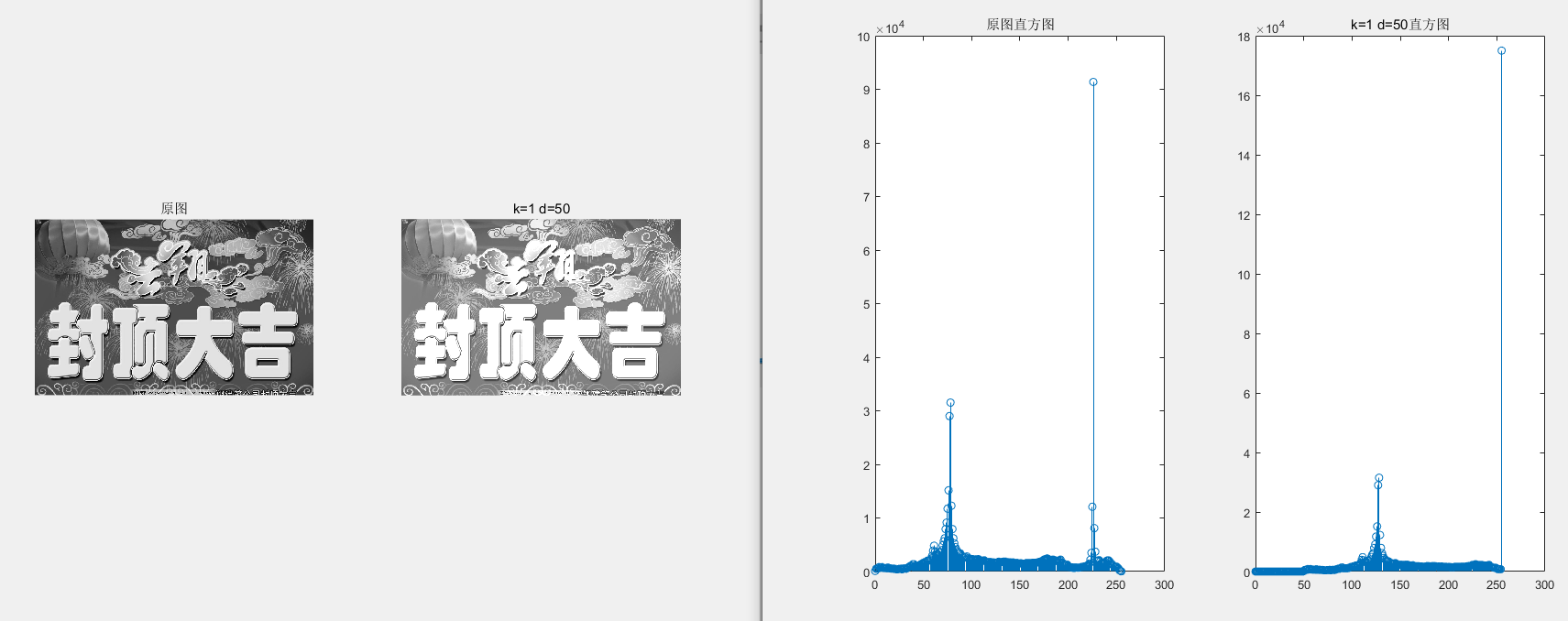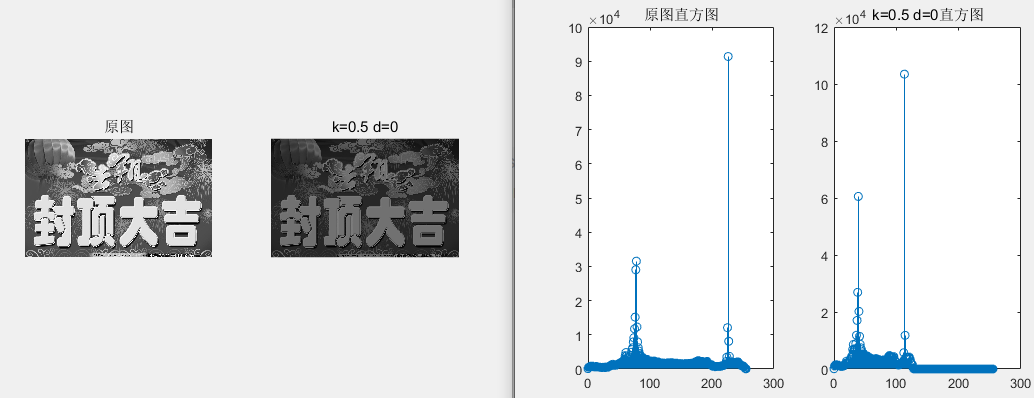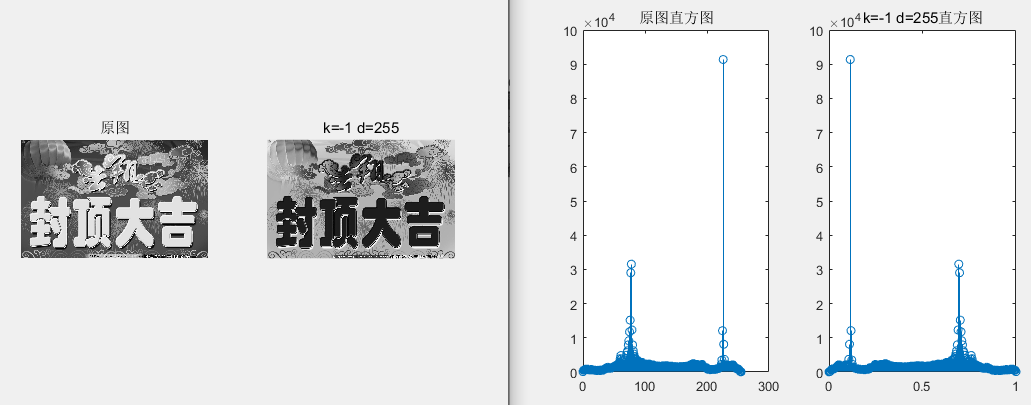7 讨论
线性变换是一个有限的查表操作，在C++实现时可以在将图像逐像素的计算过程转换为查表操作。由于灰度线性变换的查找表只需256字节，完全可以全部缓存到现代CPU的cache中，通过多线程的查表操作，可以加快整个图像的变换过程。当然，这样速度还是没有GPU中进行速度快。灰度线性变换是与相邻像素无关的操作，非常适合在GPU中并行计算。但需要根据图像大小，考虑图像从CPU到GPU再从GPU到CPU的时间损耗，时间加快只对很大的图有效。


展开全文• 前一篇文章讲解了图像灰度化处理的知识，结合OpenCV调用cv2.cvtColor()函数实现图像灰度操作，使用像素处理方法对图像进行灰度化处理。本文主要讲解灰度线性变换，基础性知识希望对您有所帮助。 1.图像灰度上移变换...
该系列文章是讲解Python OpenCV图像处理知识，前期主要讲解图像入门、OpenCV基础用法，中期讲解图像处理的各种算法，包括图像锐化算子、图像增强技术、图像分割等，后期结合深度学习研究图像识别、图像分类应用。希望文章对您有所帮助，如果有不足之处，还请海涵~
该系列在github所有源代码：https://github.com/eastmountyxz/ImageProcessing-Python
PS：请求帮忙点个Star，哈哈，第一次使用Github，以后会分享更多代码，一起加油。
同时推荐作者的C++图像系列知识：
[数字图像处理] 一.MFC详解显示BMP格式图片
[数字图像处理] 二.MFC单文档分割窗口显示图片
[数字图像处理] 三.MFC实现图像灰度、采样和量化功能详解
[数字图像处理] 四.MFC对话框绘制灰度直方图
[数字图像处理] 五.MFC图像点运算之灰度线性变化、灰度非线性变化、阈值化和均衡化处理详解
[数字图像处理] 六.MFC空间几何变换之图像平移、镜像、旋转、缩放详解
[数字图像处理] 七.MFC图像增强之图像普通平滑、高斯平滑、Laplacian、Sobel、Prewitt锐化详解
前文参考：
[Python图像处理] 一.图像处理基础知识及OpenCV入门函数
[Python图像处理] 二.OpenCV+Numpy库读取与修改像素
[Python图像处理] 三.获取图像属性、兴趣ROI区域及通道处理
[Python图像处理] 四.图像平滑之均值滤波、方框滤波、高斯滤波及中值滤波
[Python图像处理] 五.图像融合、加法运算及图像类型转换
[Python图像处理] 六.图像缩放、图像旋转、图像翻转与图像平移
[Python图像处理] 七.图像阈值化处理及算法对比
[Python图像处理] 八.图像腐蚀与图像膨胀
[Python图像处理] 九.形态学之图像开运算、闭运算、梯度运算
[Python图像处理] 十.形态学之图像顶帽运算和黑帽运算
[Python图像处理] 十一.灰度直方图概念及OpenCV绘制直方图
[Python图像处理] 十二.图像几何变换之图像仿射变换、图像透视变换和图像校正
[Python图像处理] 十三.基于灰度三维图的图像顶帽运算和黑帽运算
[Python图像处理] 十四.基于OpenCV和像素处理的图像灰度化处理
前一篇文章讲解了图像灰度化处理的知识，结合OpenCV调用cv2.cvtColor()函数实现图像灰度操作，使用像素处理方法对图像进行灰度化处理。本文主要讲解灰度线性变换，基础性知识希望对您有所帮助。
1.图像灰度上移变换：DB=DA+50
2.图像对比度增强变换：DB=DA*1.5
3.图像对比度减弱变换：DB=DA*0.8
4.图像灰度反色变换：DB=255-DA
PS：文章参考自己以前系列图像处理文章及OpenCV库函数，同时参考如下文献：
杨秀璋等. 基于苗族服饰的图像锐化和边缘提取技术研究[J]. 现代计算机，2018(10).
《数字图像处理》（第3版），冈萨雷斯著，阮秋琦译，电子工业出版社，2013年.
《数字图像处理学》（第3版），阮秋琦，电子工业出版社，2008年，北京.
《OpenCV3编程入门》，毛星云，冷雪飞，电子工业出版社，2015.
[数字图像处理] 五.MFC图像点运算之灰度线性变化、灰度非线性变化、阈值化和均衡化处理详解

一.图像灰度线性变换原理
图像的灰度线性变换是通过建立灰度映射来调整原始图像的灰度，从而改善图像的质量，凸显图像的细节，提高图像的对比度。灰度线性变换的计算公式如下所示：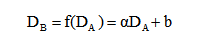该公式中DB表示灰度线性变换后的灰度值，DA表示变换前输入图像的灰度值，α和b为线性变换方程f(D)的参数，分别表示斜率和截距。

当α=1，b=0时，保持原始图像
当α=1，b!=0时，图像所有的灰度值上移或下移
当α=-1，b=255时，原始图像的灰度值反转
当α>1时，输出图像的对比度增强
当0<α<1时，输出图像的对比度减小
当α<0时，原始图像暗区域变亮，亮区域变暗，图像求补

如图所示，显示了图像的灰度线性变换对应的效果图。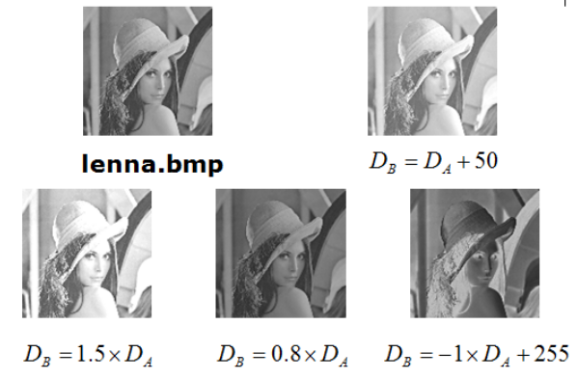二.图像灰度上移变换
该算法将实现图像灰度值的上移，从而提升图像的亮度，其实现代码如下所示。由于图像的灰度值位于0至255区间之内，所以需要对灰度值进行溢出判断。
# -*- coding: utf-8 -*-
import cv2
import numpy as np
import matplotlib.pyplot as plt

#读取原始图像

#图像灰度转换
grayImage = cv2.cvtColor(img, cv2.COLOR_BGR2GRAY)

#获取图像高度和宽度
height = grayImage.shape
width = grayImage.shape

#创建一幅图像
result = np.zeros((height, width), np.uint8)

#图像灰度上移变换 DB=DA+50
for i in range(height):
for j in range(width):

if (int(grayImage[i,j]+50) > 255):
gray = 255
else:
gray = int(grayImage[i,j]+50)

result[i,j] = np.uint8(gray)

#显示图像
cv2.imshow("Gray Image", grayImage)
cv2.imshow("Result", result)

#等待显示
cv2.waitKey(0)
cv2.destroyAllWindows()

其输出结果如下图所示，图像的所有灰度值上移50，图像变得更白了。注意，纯黑色对应的灰度值为0，纯白色对应的灰度值为255。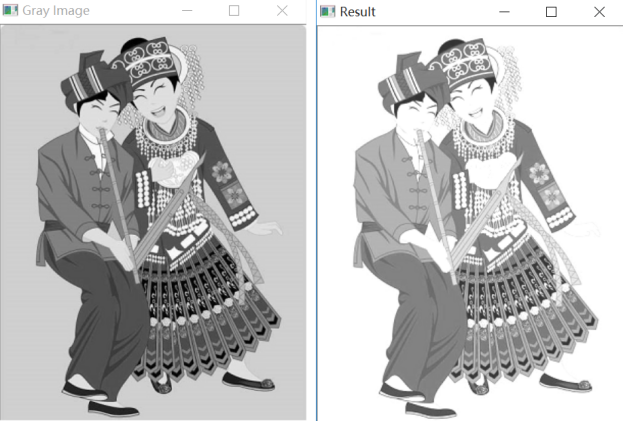三.图像对比度增强变换
该算法将增强图像的对比度，Python实现代码如下所示：
# -*- coding: utf-8 -*-
import cv2
import numpy as np
import matplotlib.pyplot as plt

#读取原始图像

#图像灰度转换
grayImage = cv2.cvtColor(img, cv2.COLOR_BGR2GRAY)

#获取图像高度和宽度
height = grayImage.shape
width = grayImage.shape

#创建一幅图像
result = np.zeros((height, width), np.uint8)

#图像对比度增强变换 DB=DA*1.5
for i in range(height):
for j in range(width):

if (int(grayImage[i,j]*1.5) > 255):
gray = 255
else:
gray = int(grayImage[i,j]*1.5)

result[i,j] = np.uint8(gray)

#显示图像
cv2.imshow("Gray Image", grayImage)
cv2.imshow("Result", result)

其输出结果如下图所示，图像的所有灰度值增强1.5倍。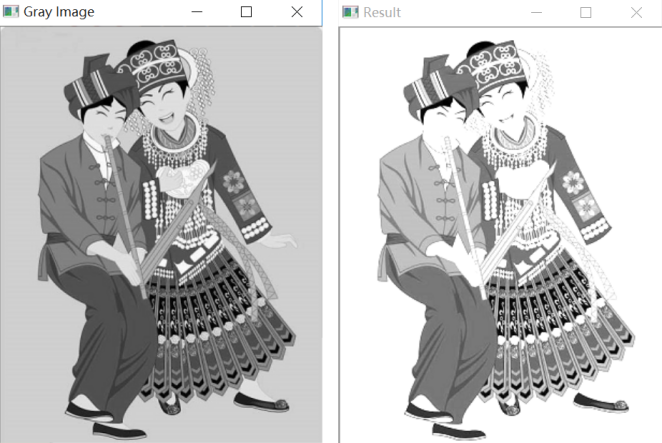四.图像对比度减弱变换
该算法将减弱图像的对比度，Python实现代码如下所示：
# -*- coding: utf-8 -*-
import cv2
import numpy as np
import matplotlib.pyplot as plt

#读取原始图像

#图像灰度转换
grayImage = cv2.cvtColor(img, cv2.COLOR_BGR2GRAY)

#获取图像高度和宽度
height = grayImage.shape
width = grayImage.shape

#创建一幅图像
result = np.zeros((height, width), np.uint8)

#图像对比度减弱变换 DB=DA*0.8
for i in range(height):
for j in range(width):
gray = int(grayImage[i,j]*0.8)
result[i,j] = np.uint8(gray)

#显示图像
cv2.imshow("Gray Image", grayImage)
cv2.imshow("Result", result)

#等待显示
cv2.waitKey(0)
cv2.destroyAllWindows()

其输出结果如下图所示，图像的所有灰度值减弱，图像变得更暗。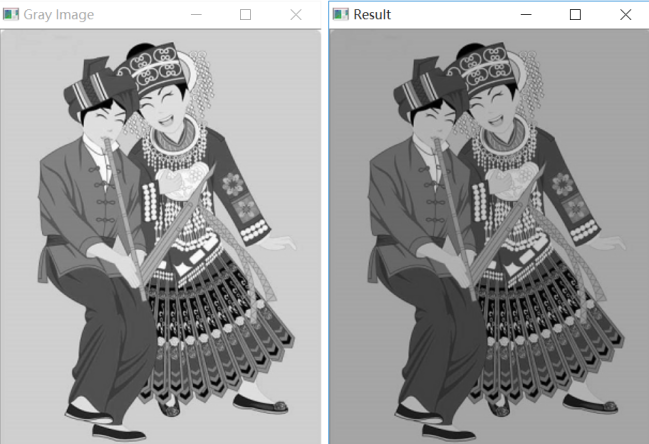五.图像灰度反色变换
反色变换又称为线性灰度求补变换，它是对原图像的像素值进行反转，即黑色变为白色，白色变为黑色的过程。其Python实现代码如下所示：
# -*- coding: utf-8 -*-
import cv2
import numpy as np
import matplotlib.pyplot as plt

#读取原始图像

#图像灰度转换
grayImage = cv2.cvtColor(img, cv2.COLOR_BGR2GRAY)

#获取图像高度和宽度
height = grayImage.shape
width = grayImage.shape

#创建一幅图像
result = np.zeros((height, width), np.uint8)

#图像灰度反色变换 DB=255-DA
for i in range(height):
for j in range(width):
gray = 255 - grayImage[i,j]
result[i,j] = np.uint8(gray)

#显示图像
cv2.imshow("Gray Image", grayImage)
cv2.imshow("Result", result)

#等待显示
cv2.waitKey(0)
cv2.destroyAllWindows()

其输出结果如下图所示，图像处理前后的灰度值是互补的。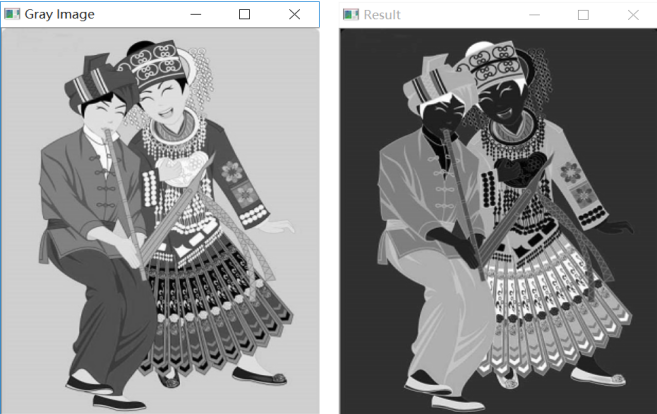图像灰度反色变换在医学图像处理中有一定的应用，如下图所示：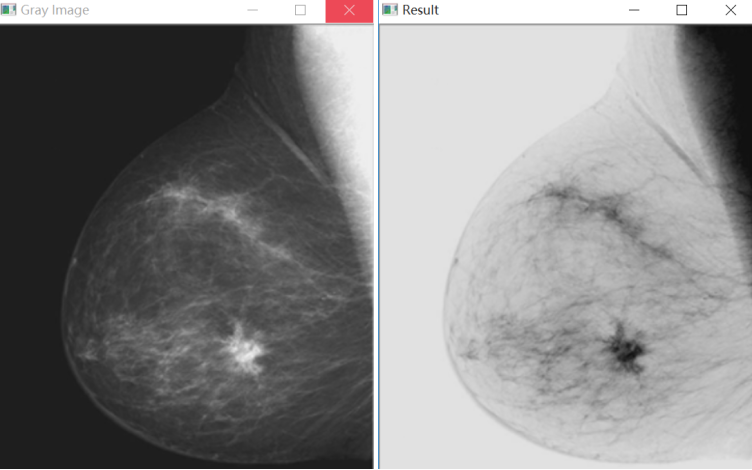希望文章对大家有所帮助，如果有错误或不足之处，还请海涵。最近连续奔波考博，经历的事情太多，有喜有悲，需要改变自己好好对女神，也希望读者与我一起加油。
（By：Eastmount 2019-03-28 深夜12点 https://blog.csdn.net/Eastmount/）


展开全文python图像处理 灰度处理 线性变换 opencv 图像处理
• 图像的灰度线性变换 图像增强：线性、 分段线性、 对数、 反对数、 幂律(伽马)变换、直方图均衡. 感谢各位博主分享，侵删 关于OpenCV和numpy模块 需要先导入cv2和numpy库，我的pycharm已经安装了anacond...
主要参考内容

数字图像处理-空间与处理-灰度变换-基本灰度变换函数（求反、线性灰度变换、换、对数变换、伽马变换、阈值化/二值化）
[Python图像处理] 十五.图像的灰度线性变换
图像增强：线性、 分段线性、 对数、 反对数、 幂律(伽马)变换、直方图均衡.
感谢各位博主分享，侵删

关于OpenCV和numpy模块

导入cv2，在anaconda中搜索py-opencv，install即可。

代码块后续完善后上传。


展开全文python opencv
• 图像灰度非线性变换 文章目录1 原理2 Matlab实现3 OpenCV实现3.1 实现3.2 注意4 效果图 1 原理   图像灰度的非线性变换主要有对数变换、指数变换、幂次变换等。本文主要讨论对数变换。   对数变换的基本形式...
• 1、分段线性变换各种情况的变换效果对比 2、对数、指数变换、取反等多种非线性变换的效果对比 变换结果与分析 分段线性变换 1）变换函数 2）分段线性变换结果 3）分段线性变换对比分析 通过变换，将灰度...图像识别
• 前一篇文章讲解了图像灰度化处理线性变换知识，结合OpenCV调用cv2.cvtColor()函数实现图像灰度操作，本篇文章主要讲解非线性变换，使用自定义方法对图像进行灰度化处理，包括对数变换和伽马变换。本文主要讲解灰度...Python图像处理 对数变换
• 前面讲过的“非0即1法”，固定阈值法，双固定阈值法等都属于非线性变换。这里再补充几种常用的非线性变换。 一、灰度对数变换  对数变换实现了图像的灰度扩展和压缩的功能。它扩展低灰度值而压缩高灰度值，让图像...图像处理 灰度对数变换
• 灰度变换图像增强的一种重要手段，用于改善图像显示效果，属于空间域处理方法，它可以使图像动态范围加大，使图像对比度扩展，图像更加清晰，特征更加明显。灰度变换其实质就是按一定的规则修改图像每一个像素的...数字图像处理 Python OpenCV
• 图像的线性变换图像处理的基本运算，通常应用调整图像的画面质量方面，如图像对比度、亮度及反转等操作。对于输入图像f(x,y)，输出图像g(x,y)，其线性变换表达式为： 其中参数a表示图像对比度变化，b表示图像...线性变换
• 图像灰度线性变换 假定原图像f(x,y)f(x,y)f(x,y)的灰度范围为[a,b]，变换后图像g(x,y)g(x,y)g(x,y)灰度扩展为[c,d],则根据线性方程式可以得到： (9-1)g(x,y)=d−cb−a[f(x,y)−a]+c g(x,y) = \frac{d - c}{b - a}[f...图像灰度变换 图像处理 数字图像处理 人工智能
• 线性变换 直观定义 几种基本线性变换与对应的矩阵表示 旋转 翻转镜像 缩放伸缩 推移错切 仿射变换 投影变换 总结平面变换包括 线性变换，仿射变换等， 线性变换包括 旋转，镜像(翻转)，伸缩（缩放），推移（错切） ...
• 灰度的线性变换 Db = f(Da) = k*Da +b k为斜率，b为y轴交点截距，Da为输入图像的灰度，Db为输出图像的灰度 三个有趣的结论，相信会对灰度变换的理解更加深刻： 1，k> 1增加对比度，k 2，k=1改变亮度 3，k=1,b=0...图像处理 matlab 测试
• 图像点处理是图像处理系列的基础，主要用于让我们熟悉Matlab图像处理的编程环境。灰度线性变换和灰度拉伸是对像素灰度值的变换操作，直方图是对像素灰度值的统计，直方图均衡是对灰度值分布的变换图像处理 matlab 线性变换 直方图均衡化
• 一.理论基础 线性灰度变换函数是一个线性函数：B=f(A)=aA+c.其中A为输入图像灰度值，B为输出图像灰度，a为线性函数的斜率，c为Y轴的截距。 当a>1时，输入图像的对比度将增大，...这种线性变换可能由于像素达到...
• 灰度变换法：  一般成像系统只具有一定的亮度范围，亮度的最大值与最小值之比称为...灰度变换法又可分为三种：线性分段性及分线性变换。 （1）线性变换  假定原图像f(x,y)的灰度范围为[a,b],希望变换后图像g(x,y)
• 转自https://blog.csdn.net/eastmount/article/details/46312145本文主要讲述基于VC++6.0 ...包括图像灰度线性变换、灰度非线性变换图像阈值化处理图像均衡化处理等知识，并结合前一篇论文灰度直方图进行展示 。...
• 其作用是当图像像素的灰度值集中一定范围如[a,b]时，可以经由线性变换公式将其映射到新的范围[c,d]，如果将c取为0，将d取为255，则映射到整个灰度级中，即最大可能的加大了图像的对比度。经过上面的分析，再由数学...图像处理 线性变换
...